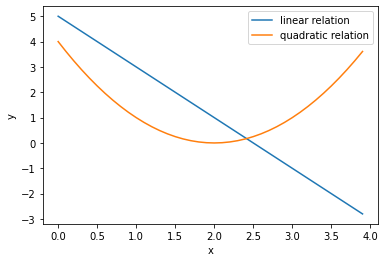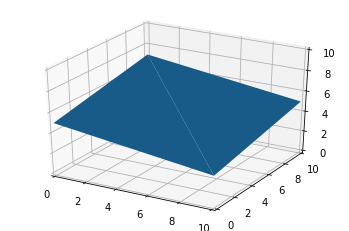# Linear Algebra and Numpy¶

Linear algebra is the math underlying much of machine learning, including sklearn's LinearRegression we've been using. You should probably take a linear algrebra course at some point if you haven't already.

In 320, we'll some very basic ideas. Let's start with some intuition.

In :
import pandas as pd
import numpy as np
import matplotlib.pyplot as plt
%matplotlib inline
plt.rcParams["font.size"] = 16


A linear relationship between two variables looks like a straight line when plotted (contrast this with the quadtratic line, which is not linear):

In :
df = pd.DataFrame({"x": np.arange(0, 4, 0.1)})
df["linear relation"] = df["x"] * -2 + 5
df["quadratic relation"] = (df["x"] - 2) ** 2
ax = df.set_index("x").plot.line()
ax.set_ylabel("y")

Out:
Text(0, 0.5, 'y')Straight lines generalize to flat planes in 3 dimensions (or hyperplanes in more dimensions!).

In :
fig = plt.figure()
ax = fig.add_subplot(111, projection='3d', xlim=(0,10), ylim=(0,10), zlim=(0,10))
ax.plot_trisurf(np.array([0, 0, 10, 10]),
np.array([0, 10, 10, 0]),
np.array([5,7,5,3]))

Out:
<mpl_toolkits.mplot3d.art3d.Poly3DCollection at 0x7fab20d716a0>## Numpy Arrays¶

Linear algebra will involve working with matrices and vectors; we'll learn numpy for that. Pandas and sklearn are already based on numpy.

In :
df = pd.DataFrame([[0,2,1], [2,3,4], [8,5,6]], columns=["x1", "x2", "y"])
df

Out:
x1 x2 y
0 0 2 1
1 2 3 4
2 8 5 6

Internally, we have a numpy array:

In :
df.values # a 2-dimensional numpy array

Out:
array([[0, 2, 1],
[2, 3, 4],
[8, 5, 6]])
In :
type(df.values)

Out:
numpy.ndarray
In :
from sklearn.linear_model import LinearRegression
lr = LinearRegression()
lr.fit(df[["x1", "x2"]], df["y"])

Out:
LinearRegression(copy_X=True, fit_intercept=True, n_jobs=None, normalize=False)
In :
lr.coef_ # a 1-dimension numpy array

Out:
array([-2.,  7.])

A 2-dimensional array is called a matrix, and a 1-dimensional array is called a vector. A tensor is a general term for an N-dimensional array. A 0-dimensional array is called a scalar, and is also possible in numpy:

In :
np.array(3.14)

Out:
array(3.14)

Reshaping an array is easy: just specify the number of rows, columns.

In :
a = np.array([[1,2,3], [4,5,6]])
a

Out:
array([[1, 2, 3],
[4, 5, 6]])
In :
b = a.reshape(3, 2)
b

Out:
array([[1, 2],
[3, 4],
[5, 6]])

Some shapes wouldn't work (e.g., a.reshape(3, 4) would fail, because a only has 6 values, but the reshape would require 12). If we pass -1 for a dimension, numpy calculates how large it should be.

In :
b = a.reshape(3, -1)
b

Out:
array([[1, 2],
[3, 4],
[5, 6]])

We can index into an array with ARRAY[ROW, COL]:

In :
b[2, 1]

Out:
6

Be careful with modifications! Even after reshape or slicing, these get reflected back to the original (in contrast, a slice of a list produces a new list object).

In :
b[2, 1] = 100
a

Out:
array([[  1,   2,   3],
[  4,   5, 100]])

You can always explicitly make a copy if necessary.

In :
c = a.copy()
c[0,0] = -3
c

Out:
array([[ -3,   2,   3],
[  4,   5, 100]])
In :
a

Out:
array([[  1,   2,   3],
[  4,   5, 100]])

## Dot Product¶

In its simplest form, the dot product in linear algebra multiplies a horizontal vector by a vertical vector. Numpy doesn't have a way to specify a vector's direction, so we'll actually encode them as matrices that have one row (horizontal) or one column (vertical).

In :
a = np.array([10, 0, 20, -1])
a

Out:
array([10,  0, 20, -1])
In :
b = np.array([2, 9, 0.5, 3])
b

Out:
array([2. , 9. , 0.5, 3. ])
In :
a = a.reshape(1, -1) # one row, however many columns necessary (horizontal vector)
a

Out:
array([[10,  0, 20, -1]])
In :
b = b.reshape(-1, 1) # one column, however many rows necessary (vertical vector)
b

Out:
array([[2. ],
[9. ],
[0.5],
[3. ]])

@ is the dot product operator in Python.

In :
a @ b

Out:
array([[27.]])

To get that, we multiplied the first number in a by the first number in b; then we multiplied the second number in a by the second number in b, and so on. At the end, we added the four products.

It was like this:

In :
10*2 + 0*9 + 20*0.5 + -1*3

Out:
27.0

The dot product is key to how sklearn's LinearRegression model as well as the classification model we'll learn in a week make predictions. We won't explain more about the following code here, but observe that lr's predictions can be computed with a simple expression using a dot product.

In :
lr.predict(df[["x1", "x2"]])

Out:
array([1., 4., 6.])
In :
df[["x1", "x2"]] @ lr.coef_ + lr.intercept_

Out:
0    1.0
1    4.0
2    6.0
dtype: float64